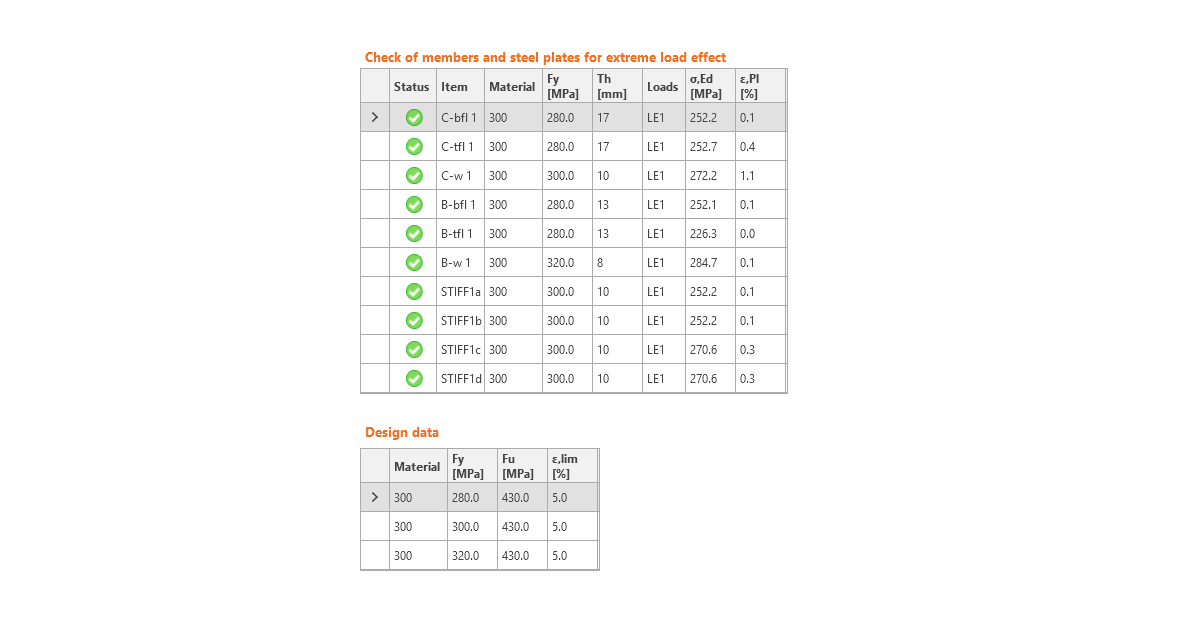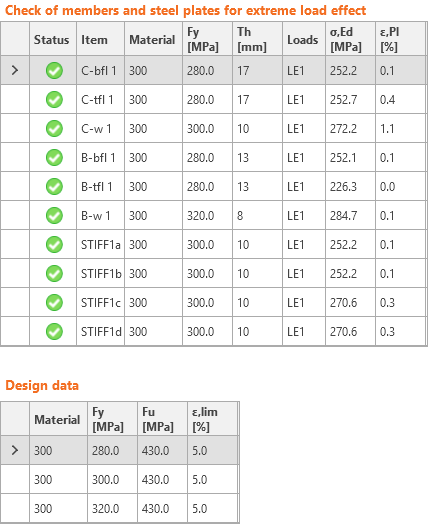### Choose language# Code-check of steel plates according to Australian standards

$$Strain check is performed at shell finite elements simulating plates. The yield strength is decreased by the capacity factor.The resulting equivalent stress (HMH, von Mises) and plastic strain are calculated on plates. When the yield strength (multiplied by capacity factor ϕ = 0.9, which is editable in Code setup) on the bilinear material diagram is reached, the check of the equivalent plastic strain is performed. The limit value of 5 % is suggested in Eurocode (EN1993-1-5 App. C, Par. C8, Note 1). This value can be modified in the Code setup, but verification studies were made for this recommended value.The plate element is divided into five layers, and elastic/plastic behavior is investigated in each of them. The program shows the worst result from all of them.The CBFEM method can provide stress a little bit higher than yield strength. The reason is the slight inclination of the plastic branch of the stress-strain diagram, which is used in the analysis to improve the stability of the interaction calculation. This is not a problem for practical design. The equivalent plastic strain is exceeded at higher stress, and the joint does not satisfy anyway.$$